Courses

# Stability Analysis of Linear Control Systems - 2

## 20 Questions MCQ Test Topicwise Question Bank for Electrical Engineering | Stability Analysis of Linear Control Systems - 2

Description
This mock test of Stability Analysis of Linear Control Systems - 2 for Electrical Engineering (EE) helps you for every Electrical Engineering (EE) entrance exam. This contains 20 Multiple Choice Questions for Electrical Engineering (EE) Stability Analysis of Linear Control Systems - 2 (mcq) to study with solutions a complete question bank. The solved questions answers in this Stability Analysis of Linear Control Systems - 2 quiz give you a good mix of easy questions and tough questions. Electrical Engineering (EE) students definitely take this Stability Analysis of Linear Control Systems - 2 exercise for a better result in the exam. You can find other Stability Analysis of Linear Control Systems - 2 extra questions, long questions & short questions for Electrical Engineering (EE) on EduRev as well by searching above.
QUESTION: 1

Solution:
QUESTION: 2

### For a discrete-time system to be unstable, all the poles of the z-transfer function should lie

Solution: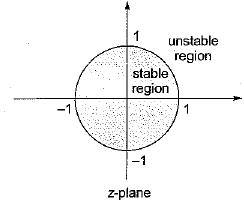QUESTION: 3

### Consider the following characteristic equation of a system: s3 + 2Ks2 + (K+ 2) s+ 4 = 0 Which one of the following is correct?

Solution:

Given characteristic equation is,
s2 + 2Ks2 + (K + 2)s + 4 = 0
Routh’s array is: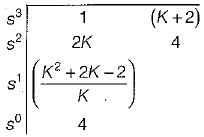For stability, 2K > 0 or K > 0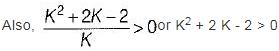Now, K2 + 2K - 2 = 0 or, K = 0.73, -2.73
For K > 0.73, K2 + 2 K - 2 > 0
(since K should be > 0)
Hence, system will be stable if K > 0.73.

QUESTION: 4

For which of the following values of K, the feedback system shown in the below figure is stable?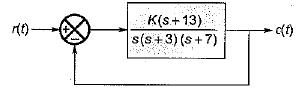Solution:

The characteristic equation is
1 + G (s) H (s) = 0
or,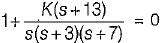or, s3 + 10s2 + (21 + K )s + 13 K= 0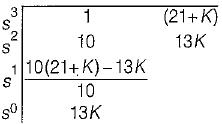For stability, 13K > 0 or K > 0
Also,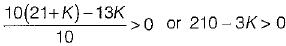or, K < 70
Hence, 0 < K< 70 (For stability).

QUESTION: 5

What is the range of K for which the open loop transfer function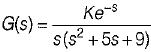represents an unstable closed loop system?

Solution:

For low frequency, e-s = (1 - s)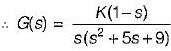The characteristic equation is
1 + G(s) = 0
or, s(s2 + 5s + 9) + K(1 - s) = 0
or, s3 + 5s2 + (9 - K) s + K = 0
The Routh’s array is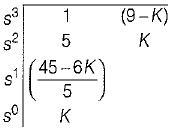For stability, K > 0 and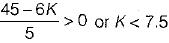∴ 0 < K < 7.5 (for stability)
0 > K > 7.5 (for unstability)

QUESTION: 6

What is the range of values of K (K > 0) such that the characteristic equation
s3 + 3 (K + 1)s2 + (7K + 5)s + (4K + 7) = 0
has roots more negative than s = -1?

Solution:

Putting s = (z - 1) in the given characteristic equation, we get:
(z - 1)3 + 3 (K + 1) (z - 1)2 + (7K+ 5) (z - 1) + (4K + 7) = 0
or, z3 + 3 Kz2 + (K + 2)z + 4 = 0
Routh’s array is: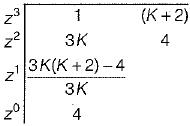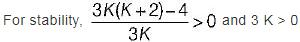i.e. 3K2 + 6K - 4 > 0 and K > 0
Now, roots of 3K2 + 6K - 4 = 0 are: K = -2.5, 0.53
Since K > 0 ∴ K = 0.53 (K = -2.5 neglected)
For K > 0.53, 3 K2 + 6 K - 4 > 0
Hence, the range of value of K is
0.53 < K < ∞ or ∞ > K > 0.53

QUESTION: 7

A closed loop control system is shown below: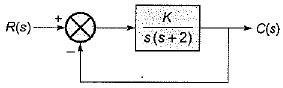Match List - I (Different values of K) with List - II (Roots of Characteristic Equation) and select the correct answer using the codes given below the lists: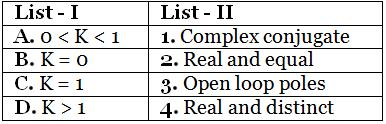Codes: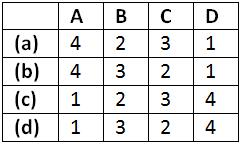Solution:

The characteristic equation is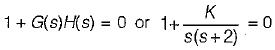or, s2 + 2 s + K = 0
or,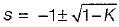When, 0 < K < 1, roots are real and distinct.
When, K = 0, roots are s = 0, -2 (Poles of open loopT.F. G(s)H(s)).
When, K = 1, roots are s = -1, -1 i.e. real and equal.
When, K > 1, roots are complex conjugate.

QUESTION: 8

Consider the following statements regarding the characteristic equation of a system given by:
s4 + 5s3 + 25+10 = 0
1. The system is unstable.
2. The system is stable.
3. Number of roots with zero real part - 0
4. Number of roots with positive real part - 4
5. Number of roots with negative real part = 2
Which of the above statements are correct?

Solution:

The Routh’s array is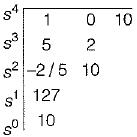Since there are two sign changes in first column of Routh’s array, therefore the system is unstable.
Number of roots with positive real part = 2
Number of roots with negative real part = 2
Number of roots with zero real part = 0

QUESTION: 9

The transfer function of a system is (1 + 2s)/(1 - s)
The system is then which one of the following?

Solution:

Since one pole (s = 1) lies in RH s-plane, therefore the system is unstable.

QUESTION: 10

Assertion (A): For a system to be stable, the roots of the characteristic equation should not lie in the right half of the s-plane or on the imaginary axis.
Reason (R): A system is said to be stable if the zeros of the closed loop transfer function are all in the left half of s-plane.

Solution:

Poles of the closed loop transfer function is the characteristic equation of a closed loop control system. For the system to be stable all roots of characteristic equation should lie in LH s-plane. Hence reason is false.

QUESTION: 11

Assertion (A): Location of the poles of a transfer function in the s-plane affects greatly the transient response of the system and hence stability.
Reason (R): The poles that are close to the imaginary axis in the left-half s-plane give rise to transient responses that will decay relatively slowly, whereas the poles that are far away from the axis (relative to the dominant poles) correspond to fast-decaying time responses.

Solution:
QUESTION: 12

Assertion (A): Routh-Hurwitz criterion doesn’t provides the information about the number of roots that lie on the jω-axis while it tests whether any of the roots of the characteristic equation lie in the right-half s-plane or not.
Reason (R): The Routh-Hurwitz criterion represents a method of determining the location of zeros of a polynomial with constant real coefficients with respect to the left half and right half of the s-plane, without actually solving for the zeros.

Solution:

Routh-Hurwitz criterion tests whether any roots of the characteristic equation lie in the right-half s-plane as well as the number of roots that lie on the jω-axis.. Therefore, assertion is false.

QUESTION: 13

Consider the following statements related to Routh-Hurwitz criterion of determining the stability of a system:
1. It is applicable if the characteristic equation has real coefficients, complex terms or exponential functions of s.
2. The criterion can be applied to any stability boundaries in complex plane, such as the unit circle in the z-plane.
3. The number of changes of signs in the elements of the first column equals the number of roots with negative real parts.
Which of these statements is/are not correct?

Solution:

• Routh-Hurwitz criterion is not applicable if characteristic equation has coefficients which are exponential or complex.
• It is not applied in z-plane because z-plane having unit circle is the stability boundary of discrete-data system.
• Statement-3 is also false because no. of sign change in 1st column of Routh’s array indicates the number of roots with positive real part or roots lying in RH s-plane.

QUESTION: 14

The characteristic equation of a linear discrete-data control system is given as:
F(z) = z3 + z2 + z + K = 0,
Where K is a real constant.
What is the range of values of K so that the system is stable?

Solution:

Given, F(z) = z3 + z2 + z + k = 0
Using bilinear transformation, we put z =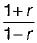in the above characteristic equation.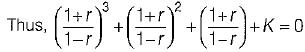or, (1 - K)r3 + (1 + 3K) r2 + 3(1 - K)r + 3 + K = 0
Routh’s array is: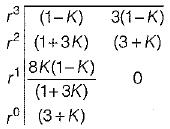For the system to be stable, we have:
1 - K > 0 or K < 1    ...(i)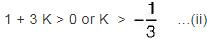3 + K > 0 or K > - 3    ..(iii)
From equation (i), (ii) and (iii) the range of values of K for stability is, 0 < K < 1.

QUESTION: 15

The characteristic equation of a linear time-invariant discrete-data system is given by:
F(z) = anzn + an - 1 zn - 1 + .......... + az + a0 = 0
where all the coefficients are real.
According to “Jury’s stability criterion”, there are certain necessary conditions which must be satisfied for the above discrete-data system to have no roots on or outside the unit circle.
Which of the following necessary condition does not holds true for the discrete-data system to have all roots inside the unit circle according to “Jury's stability criterion"?

Solution:

The necessary conditions for stability are: F (1) > 0
F(-1) > 0, if n = even integer
F(-1) < 0, if n = odd integer
and |a0| < |an|

QUESTION: 16

The first two rows of Routh’s tabulation of a third-order system are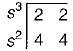Select the correct answer from the following choices.

Solution:

Routh’s array is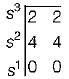Since Routh’s array ends abruptly, therefore we construct auxiliary equation for finding other elements of Routh’s array.
A2(s) = 4s2 + 4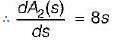Hence, new Routh’s array is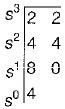Since there is no sign change in 1st column of Routh’s array, therefore given system will be marginally stable provided there is no repeated poles on jω - axis.
Thus, A2(s) = 0 or 4s2 + 4 = 0
or, s = ± j (distinct imaginary roots)
Hence, the given system is marginally stable having one root in LH s-plane and two roots on the jω - axis at s = ± j.

QUESTION: 17

The characteristic equation of a second order discrete-data system is given by:
F(z) = z2+ z+ 0.25 = 0
The above system is

Solution:

For a second order discrete- data system given by:
F(z) = a2z2 + a1z + a0 = 0
to be stable, the necessary and sufficient conditions are:
F(1) > 0
F(-1) > 0 and |a0| < a2
Here, F(z) = z2 + z + 0.25
So,  a0 = 0.25, a1 = 1, a2 = 1
Thus, F(1) - 12+ 1 + 0.25 = 2.25 > 0
F(-1) = 1 -1 + 0.25 = 0.25 > 0
and la0l = 0.25 < a2 = 1
Since ail the conditions are satisfied, therefore given system is stable.

QUESTION: 18

The z-transform of a signal is given by: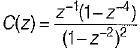Its final value is

Solution:

Given,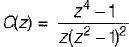Applying final value theorem,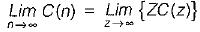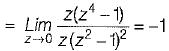QUESTION: 19

The dynamic response of a system is given by the following differential equation: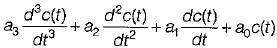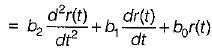where c(t) and r(t) are respectively the output and the input and a, a, a1 a0, b2, b1 and b0 are constants. It is a

Solution:
QUESTION: 20

The transfer function of a system is: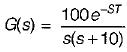The system

Solution: